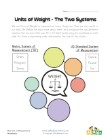Whenever we count or measure something, we always come across units. We call them Unit of Measurements, a quantity used as a standard of measurement. We measure a lot of different things, therefore we have many different kinds of units. The collection of free units worksheets is designed to help kids learn about the units of area, length, volume, weight and time. Many of these worksheets will expose students to both the Metric and Standard (Imperial) systems so they are learning both types of unit systems.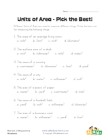Area Units - Which Works Best?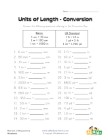Converting Units of Length Worksheet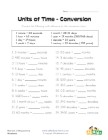Converting Units of Time Worksheet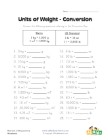Converting Units of Weight Worksheet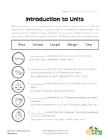Introduction to Units of Measurement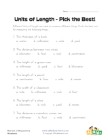Length Units - Which Works Best?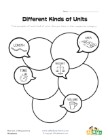Types of Units Worksheet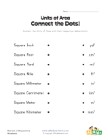Units of Area Abbreviations Worksheet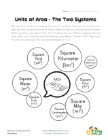Units of Area Worksheet - Metric vs. Standard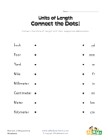Units of Length Abbreviations Worksheet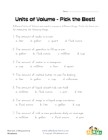Units of Volume - Which Works Best?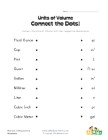Units of Volume Abbreviations Worksheet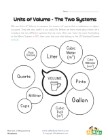Units of Volume Worksheet - Metric vs. Standard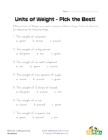Units of Weight - Which Works Best?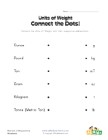Units of Weight Abbreviations Worksheet